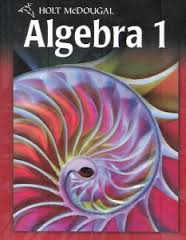# 5-2 Solving Systems By Substitution

7 Questions | Attempts: 220SettingsAlgebraic equations are not so hard to solve. When solving algebraic equations the substitution method is one the most commonly used and easiest methods when compared to the others. Test your knowledge of the substitution method by answering the questions below in preparation for your continuous assessment test. All the best as you tackle them.

• 1.
#6, p. 340
• A.

(3, 8)

• B.

(2, 3)

• C.

(─4, 4)

• D.

Not here

• 2.
#8, p. 340
• A.

(─1, 2)

• B.

(─5, ─2)

• C.

(2, 8)

• D.

Not here

• 3.
#10, p. 340
• A.

(4, 2)

• B.

(8, 0)

• C.

(0, 4)

• D.

Not here

• 4.
#2, p. 340
• A.

(4, ─10)

• B.

(─2, 8)

• C.

(18, ─52)

• D.

Not here

• 5.
#4, p. 340
• A.

(10, 0)

• B.

(12, 1)

• C.

(14, 2)

• D.

(16, 3)

• 6.
#12, p. 340
• A.

(10, 7)

• B.

(8, 6)

• C.

(6, 5)

• D.

(4, 4)

• 7.
#22, p. 340
• A.

(2, 2.5)

• B.

(5, 1)

• C.

(1, 3)

• D.

(7, 0)

## Related TopicsBack to top
×

Wait!
Here's an interesting quiz for you.